Next: The Poincaré section Up: The chaotic pendulum Previous: Numerical solution

## Validation of numerical solutions

Before proceeding with our investigation, we must first convince ourselves that our numerical solutions are valid. Now, the usual method of validating a numerical solution is to look for some special limits of the input parameters for which analytic solutions are available, and then to test the numerical solution in one of these limits against the associated analytic solution.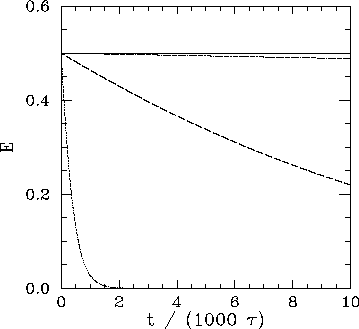One special limit of Eqs. (81) and (82) occurs when there is no viscous damping (i.e.,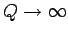) and no external driving (i.e.,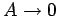). In this case, we expect the normalized energy of the pendulum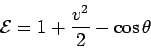(94)

to be a constant of the motion. Note that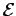is defined such that the energy is zero when the pendulum is in its stable equilibrium state (i.e., at rest, pointing vertically downwards). Figure 26 showsversus time, calculated numerically for an undamped, undriven, pendulum. Curves are plotted for various values of the parameter Nacc, which, in this special case, measures the number of time-steps taken by the integrator per (low amplitude) natural period of oscillation of the pendulum. It can be seen that for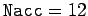there is a strong spurious loss of energy, due to truncation error in the numerical integration scheme, which eventually drains all energy from the pendulum after about 2000 oscillations. For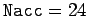, the spurious energy loss is less severe, but, nevertheless, still causes a more than 50% reduction in pendulum energy after 10,000 oscillations. For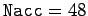, the reduction in energy after 10,000 oscillations is only about 1%. Finally, for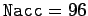, the reduction in energy after 10,000 oscillation is completely negligible. This test seems to indicate that when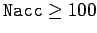our numerical solution describes the pendulum's motion to a high degree of precision for at least 10,000 oscillations.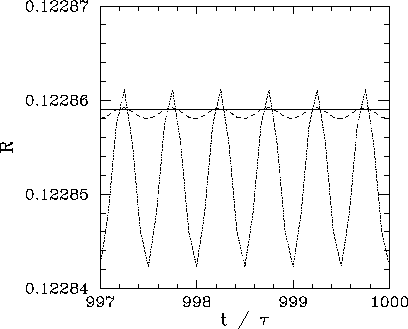Another special limit of Eqs. (81) and (82) occurs when these equations are linearized to give Eqs. (84) and (85). In this case, we expect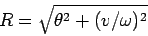(95)

to be a constant of the motion, after all transients have died away (see Sect. 4.2). Figure 27 showsversus time, calculated numerically, for a linearized, damped, periodically driven, pendulum. Curves are plotted for various values of the parameter Nacc, which measures the number of time-steps taken by the integrator per period of oscillation of the external drive. As Nacc increases, it can be seen that the amplitude of the spurious oscillations in, which are due to truncation error in the numerical integration scheme, decreases rapidly. Indeed, for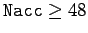these oscillations become effectively undetectable. According to the analysis in Sect. 4.2, the parametershould take the value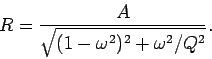(96)

Thus, for the case in hand (i.e.,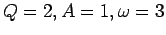), we expect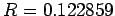. It can be seen that this prediction is borne out very accurately in Fig. 27. The above test essentially confirms our previous conclusion that when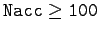our numerical solution matches pendulum's actual motion to a high degree of accuracy for many thousands of oscillation periods.Next: The Poincaré section Up: The chaotic pendulum Previous: Numerical solution
Richard Fitzpatrick 2006-03-29